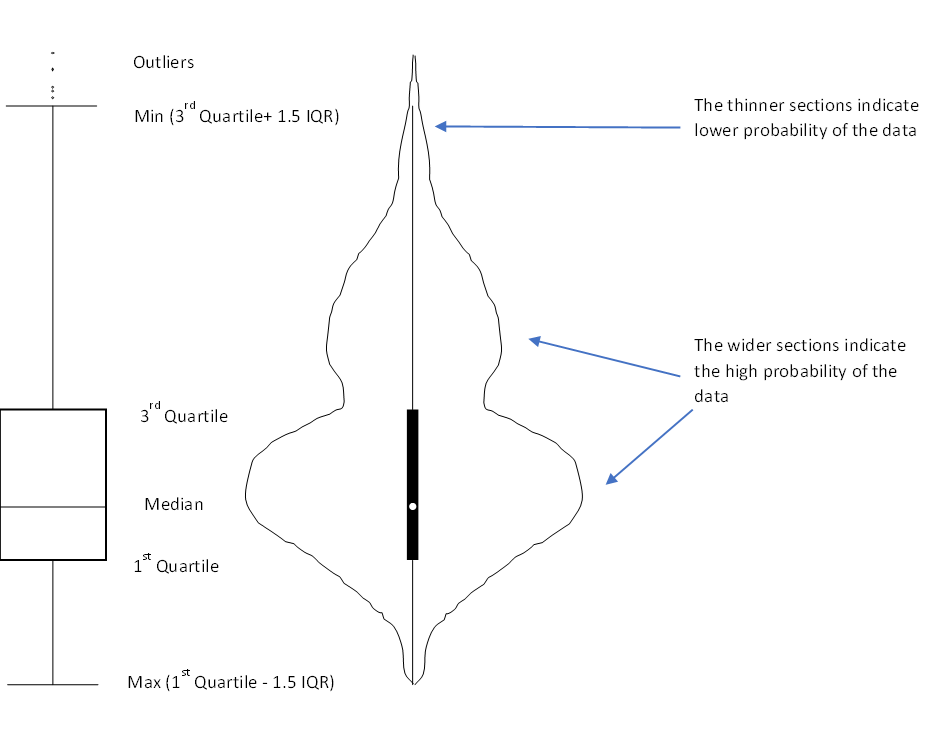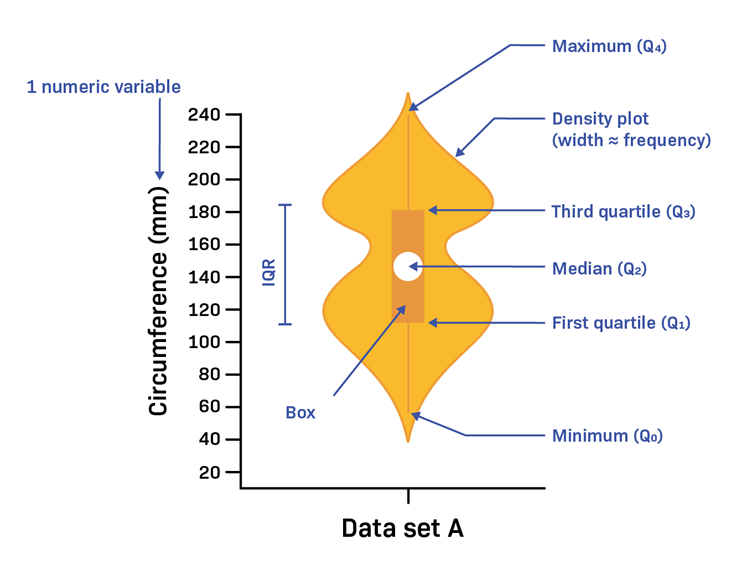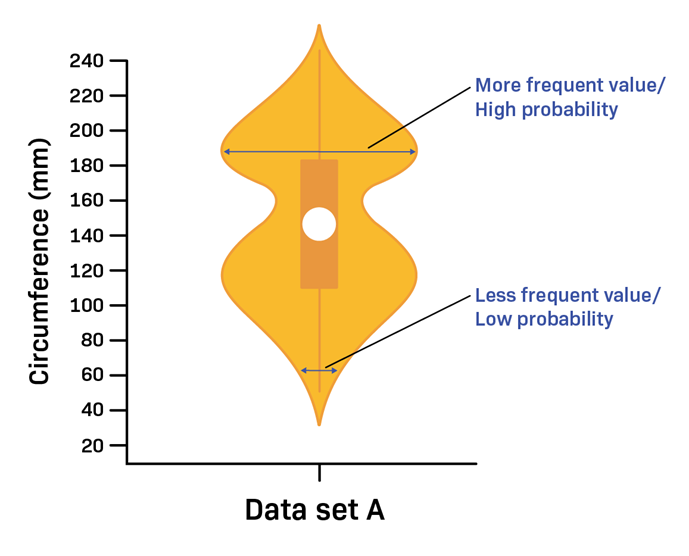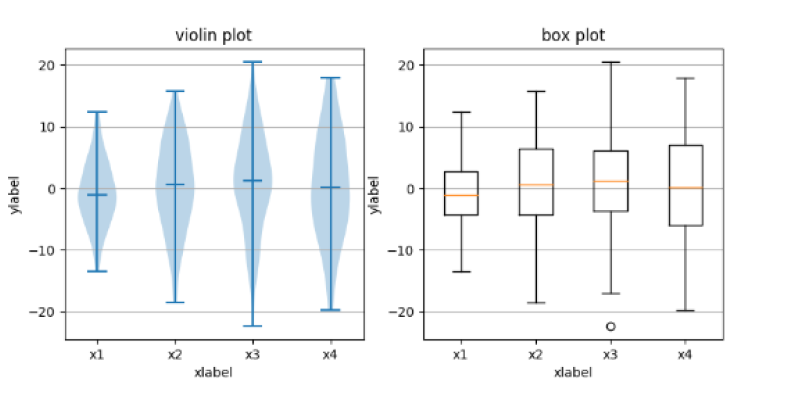• 0

# Violin Plot

Violin Plot is a graphical tool that is used to visualize both the distribution of the data and its probability density. It is similar in structure to a box plot with the addition of density plot on top of it.

An application-oriented question on the topic along with responses can be seen below. The best answer was provided by Dheeraj Bhardwaj and Anupam Goswami.

Applause for all the respondents - Vikas Choudhary, Dheeraj Bhardwaj, Suresh Kumar Gupta, Anupam Goswami, Balaji Loganathan, Dr. Babita Mallick.

## Question

Q 534. Violin plot is an extension of the regular box plot. What is a violin plot? Under which situations will you prefer to use a Violin plot instead of a Box plot?

Note for website visitors - Two questions are asked every week on this platform. One on Tuesday and the other on Friday.

• 0

# Violin Plot

This is a way of plotting numerical data which is a combination of box plot and kernel density plot. Like a box plot, this too shows the median (indicated by a white dot), the interquartile range (indicated by the broad black bar running along the plot), the minimum/ maximum (indicated by the thin black line running along the plot) and the outliers.However, on top of the above summary statistics, the violin plot also shows the data distribution which is especially preferred if the data has multiple modes (reference to the shape of violin). This allows us to see the distribution of the data and especially useful if we want to compare multiple groups.

In the above diagram, the violin plot has 2 wide sections showing that majority data points are grouped around that value. So for example if we want to study the grades obtained by students where there are generally multiple groups or modes (say Grades A and C), the violin plot is better to visualize and compare the data. Another example is if we want to compare heights of people across countries, then again, the violin plot is better. For plot of each country, we would typically observe 2 peaks (for males and females)

##### Share on other sites

• 1

Violin Plot is combination of a box plot and kernel density plot which shows peaks in data. Box Plot only provide summary statistics, Violin plot depict summary statistics and the dencity of each variable.

Before discuss Violin plot, lets discuss Box plot and Kernel density plot

Box Plot - also known as a box and whisker diagram, is a basic graphic tool that displays centering, spread, and distribution of a continous data set wherein the box represents the middle 50% of the data. Box plot is used to compare the dispersion and central tendency of data segments based on segmentations factors.

Kernel density plot represent the distribution of values in a dataset using one continuous curve. A kernel density plot is approximate to a histogram, but its better at displaying the shape of a distribution since it isn't affected by the number of bins used in the histogram.

Violin Plots contains many of the same summary statistics as box plots.

• The white dot represents the median.
• the thick gray bar in the center provide information about the interquartile range.
• the thin gray line provide information about the rest of the distributions, except for points that are determinded to be "outliers" using a method that is a function of the interquartile range.

every each side of the gray line represents kernel density estimation to show the distribution shape of the data. There wider section of the violin pivot represent a higher probability the members of the population will take on the given value; the skinnier sections represents a lower probability.

Violin Plot is preferable to box plot when you want to observe the distribution of numeric data and are especially useful when anyone want to make a comparison of distributions between multiple groups.

##### Share on other sites

• 0

A violin plot, which depicts data peaks, is a cross between a box plot and a kernel density plot. It is used to show how numerical data is distributed. Violin plots provide summary statistics as well as the density of each variable, unlike box plots, which can only show summary statistics.
The benefit of a violin plot is that it can reveal subtleties in the distribution that a boxplot cannot.

##### Share on other sites

• 0
A violin plot is a mixture of a box plot and a kernel density plot that displays the peaks in the data. Violin plot is used to visualize the distribution of numerical data and is more informative than a plain box plot since a box plot only shows summary statistics such as mean/median and interquartile ranges whereas violin plots depict summary statistics and the density of each variable.
Whenever you want to observe the distribution of numeric data or make a comparison of distributions over multiple groups Violin plots are preferred over Box Plot. The peaks, valleys, and tails of each group's density curve in Violin plot can be compared to see which groups are similar or different.
##### Share on other sites

• 0

A violin plot is a fusion of a box plot and a kernel density plot, which displays peaks in the data. It helps us to visualize the distribution of numerical data. Box plots can only show summary statistics, but violin plots can depict summary statistics and the density of each variable.

The width of the Probability Density Function defines how often that value occurs in the data set. The wider areas of the density plot display values that occur more frequently. Violin plots are also useful in multiple distributions at once for comparison.

A narrower density plot indicates values that occur less frequently. The picture below shows the shape of a data set, a violin plot can summarize a data set using five values by the following:
1.    The minimum
2.    First quartile
3.    Median
4.    Third quartile
5.    Maximum

How do you read a violin plot?

A violin plot shows how a data set varies along one variable by combining a boxplot with a Probability Density Function (PDF) the boxplot summarizes the center and spread: The white spot in the center of the box denotes the median. The length of the box indicates the (IQR) interquartile range##### Share on other sites

• 0

A Violin plot is a amalgam of a box plot and a kernel density plot that depicts peaks in the data. It is more informative than a plain box plot. A box plot only shows summary statics such as mean/median and interquartile ranges while violin plot presents the full distribution of data including density and statistics of each variable. This is very useful in cases where the data distribution is multimodal. Violin plot are useful while making a comparison of distributions between multiple groups and one can compare the peaks, valleys, and tails of each group's density curve. It intuitively shows the distribution of data in a data set. The violin plot helps in easy visualization with a viewer to understand the probability of things and therefore it is imperative for one to use a violin plot if you have a large enough dataset to capture the underlying probabilities of your dataset. But in situations where the data set is not big enough with unimodal distribution and additional information within do not add much information to show the audience which might not understand the essence of densities like in violin plot, then simple Box plot will be better suited.##### Share on other sites

• 0

There are 2 answers that stand out -

Dheeraj Bhardwaj - for explaining the two underlying plots i.e. box plot and kernel density plot

Anupam Goswami - for providing insights into where Violin Plot can be used along with examples.

Both the answers have been selected as winners. Congratulations to the joint winners!

## Create an account

Register a new account

• ### Who's Online (See full list)

• There are no registered users currently online
• ### Forum Statistics

• Total Topics
3.1k
• Total Posts
15.8k
• ### Member Statistics

• Total Members
54,460
• Most Online
990

×
×
• Create New...